# Fun Topics in Elementary Geometry

Here is a collection of fun topics more or less related to things we've done in class. Enjoy!

## Logo drawing of a 9-pointed star ¶

We used the walking-and-turning method to observe that a person who walks around the edges of a convex polygon makes exactly 360° of rotation. With this knowledge, we calculated the interior angles in a regular n-gon for various n. But we can use a related idea to understand the interior angles in a star shape. The key idea is that if you were to walk around the edges of a star, you would make two full turns! The total amount of turning is the sum of the exterior angles, so for a regular 9-pointed star, each exterior angle is 80° (80° = 720° ÷ 9). This means each interior angle is 100°. Below is a drawing, using the Pappert Logo emulator:

Using the same reasoning, it's easy to make a 13-pointed star, or to make a star which involves three full revolutions -- try it out!

At lunch one day, I was telling some friends about our class discussion of drawing a Venn diagram showing several different types of quadrilaterals: trapezoids, parllelograms, kites, rhombuses, rectangles, and squares.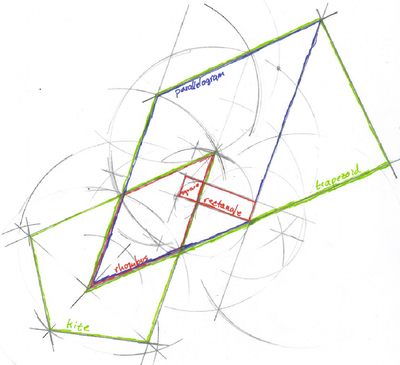Venn diagram showing relationships among quadrilaterals. Big version.

Someone remarked that I could make the problem even harder by requiring that you use an example of each shape to represent the set of those shapes! This was mostly a frivolous suggestion, but I realized there's something just slightly interesting about it: can you get the intersection of a rhombus and a rectangle to be a square? And then I drew this picture. For fun, I constructed all of the shapes using the compass and straight-edge techniques we've also learned in class.

Note that there is a boring way to do this too: you could use a square for each shape, since a square satisfies the definition of every one of the quadrilaterals on our list. The drawing above is more interesting, I think :)

## The moduli space of quadrilaterals ¶

A moduli space for a bunch of things is an object with the property that every point on that object corresponds to one of the things, and moving through the space gives you a continuously changing family of the things. For example, the moduli space of squares is the line $$(0,\infty)$$: every point on the line corresponds to a square (with that side length), and moving along the line moves through all possible squares.

Now can you visualize the moduli spaces for other quadrilaterals? If so, can you see how they fit together !? Here are pictures showing the moduli spaces of parallelograms and trapezoids!

### Parallelograms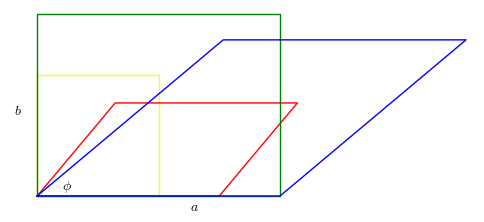The colored parallelograms above correspond to points with the same color below. The red plane contains the parallelograms with b = 1, and the purple line shows rhombuses with a = b = 1.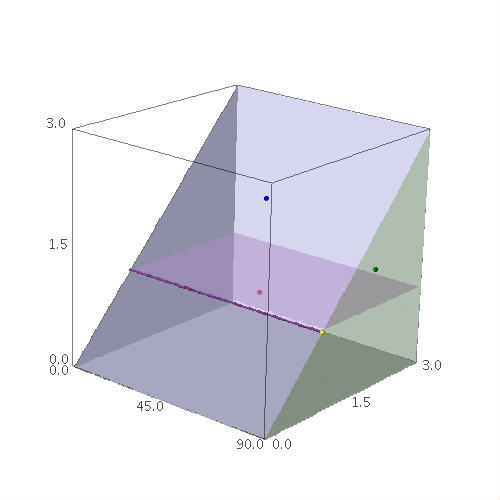Above: Parallelograms with side lengths ba, and interior angle 0° < phi ≤ 90°. The green face, where phi = 90° contains all rectangles. The blue face, where a = b, contains all rhombuses. The squares lie on their intersection.

### Trapezoids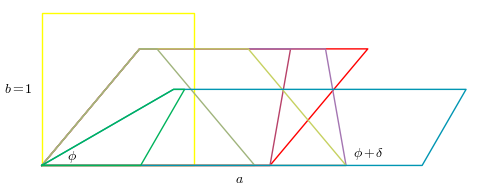The colored trapezoids above correspond to points of the same color below.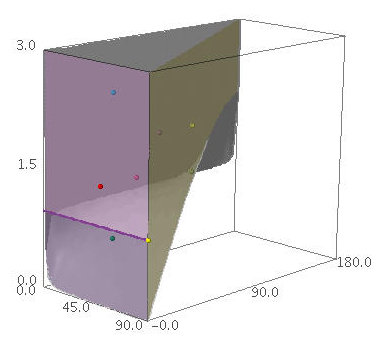Above: Trapezoids with a fixed side lengths a and b = 1, interior angle 0° < phi ≤ 90°, and exterior angle phi + delta where 0° ≤ delta ≤ 180° - 2*phi. Note that the side length a is constrained by the cosine of phi. The curved gray surface is where trapezoids with small a become triangles. The yellow plane, where phi+delta = 180° - phi contains isosceles trapezoids. The red plane, where delta = 0° and a ≥ b = 1, contains the parallelograms, and is the same red plane shown in the previous pictures. The purple line is where a = 1.

## A hypercube ¶

The hypercube is a 4-dimensional shape constructed similarly to the way a cube is constructed. In fact, if you imagine making a cube by pushing a square in the direction of the third dimension, then you understand how to make a hypercube--just push the cube in the fourth dimension.

This is the natural extension of our understanding of one, two, and three dimensions, and also comes up if you spend a while thinking about the moduli problem above. Below is a picture of the hypercube; follow the link for an animated gif which demonstrates pushing into dimensions 1, 2, 3, and 4.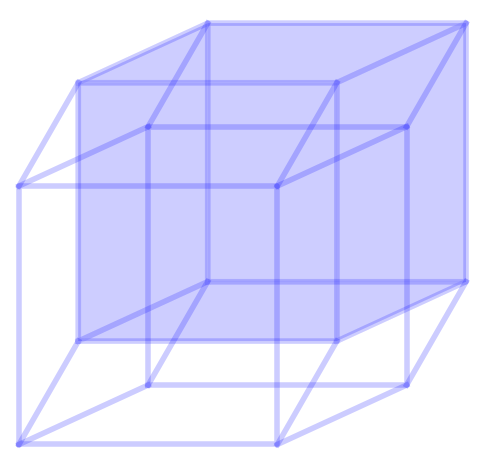A hypercube. Click for the animated gif.

This image and the accompanying animated gif were created with the open source mathematics software Sage. Here is a Sage worksheet file which generates these images.

For further reading, I recommend Tom Banchoff's essay on Drawing Cubes, part of a series on dimension. He describes different ways of drawing a cube, and at the end discusses how to use those ideas to draw a hypercube in various positions, with pictures!

## Big, BIG numbers ¶

1 00000 00000 00000 00000 00000 00000 00000 00000 00000 00000 00000 00000 00000 00000 00000 00000 00000 00000 00000 00000

That's a googol, 10^100. It's a number larger than the number of atoms in the observable universe–way larger.

A googolplex is 10^(10^100). To write down all the digits in a googolplex, you would have to write down a one followed by 10^100 zeroes. In class we calculated how long this would take: it takes about 45 seconds to write down 100 zeroes. At that rate, it would take about 450000000 seconds to write down a billion zeroes -- that's 14.3 years. So the number of years to write down all the digits in a googolplex would be 1.4 × 10^(91). For contrast, the age of the universe is estimated to be 13.75 billion years, which is about 1.4 × 10^7 years. That's tiny compared with the numbers we've been talking about.

Now of course it's easy to imagine bigger and bigger numbers by taking more powers of 10. It's not so easy to imagine bigger and bigger prime numbers. Well, of course there are infinitely many, so it's clear that bigger and bigger primes exist, but it's not so easy to think of a pattern for producing them. In fact, it's extremely difficult, and is related to a lot of interesting research in mathematics.

As of 2008, the largest known prime is 2^(43112609)-1. That's around 3.2 × 10^(10^7), which makes it more than a googol, but less than a googolplex. Just verifying the number is prime requires clever theoretical research, specialized computer programs, and many days on a supercomputer.

## Digits of pi ¶Since all circles are similar, the ratio circumference/diameter is a constant, the same for every circle, ever. This number, being such a fundamental property of circles, is of course famous. It's usually represented by the Greek letter π, pronounced "pi".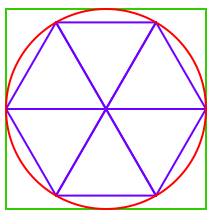3 ≤ π ≤ 4

Knowing this number is powerful knowledge then. If you know the diameter of a circle, and this number π, then you can calculate the circumference by multiplying. We saw in class that there are easy ways to get simple estimates for π. The picture at right, for example, shows that π is between 3 and 4. If you only knew 1 significant digit of π, then you would be able to calculate the circumference of a circle from its diameter accurately to 1 significant digit. Realistically, this is good enough for many purposes. The more digits of π you know, the more accurate your calculation would be.

Suppose you wanted to calculate the circumference of a circle whose diameter is 4 × 10^25 meters (the approximate diameter of the observable universe). How many digits of π would you need to know in order to have error less than 10^(-12) meters (the approximate width of a hydrogen atom)? Well, 25 + 12 is 37. Since we're multiplying by 4, the next two digits can also contribute to the 37th digit of our answer, so 39 digits of π would suffice! Surprisingly few, don't you think? (Of course, you'd also need as many significant digits in your diameter measurement!) Here are the first 40 digits of π:

3. 14159 26535 89793 23846 26433 83279 50288 4197

## Volume of a sphere ¶

Archimedes calculated the volume of a sphere by comparing it with a cylinder and two cones. Compare the slice at height h inside the sphere with the annulus at height h lying between the cones and the cylinder. Using the Pythagorean theorem one can see that each pair of slices have the same area, and therefore the volume of the sphere is equal to the difference between the volume of the cylinder and that of the two cones! This explains why the volume formula for the sphere has a factor of 4/3. from

The animation below shows these slices.

The animation was created with the free mathematics software Sage.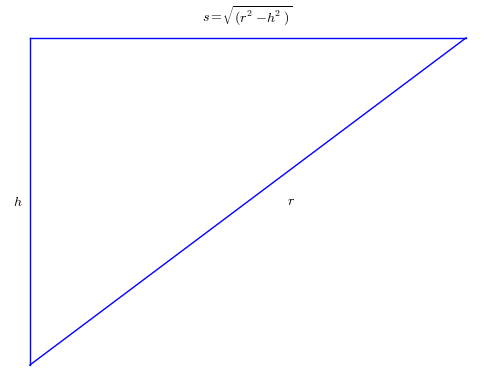This triangle, also shown in the video, gives a hint of how to understand the radius of the disk-shaped slices of the sphere. We let r denote the radius of the sphere, and use the Pythagorean theorem to express the radius of the disk at height h in terms of h and r. Now do the same for the annulus, and compare the two areas.

## Parallel lines and nonzero curvature ¶

The parallel postulate is a description of how parallel lines behave on a plane -- that is, on a 2-dimensional flat space. In class we mentioned that straight lines on a curved space can behave differently. On a sphere, for instance, every pair of straight lines cross somewhere. The straight lines are circles on the sphere which, if they were elastic, wouldn't naturally shrink to smaller circles. On the surface of the Earth, something like the arctic circle would not be a straight line, because it would shrink up to the North pole. Something like the equator or a line of longitude would be straight, because trying to tighten them wouldn't cause them to move at all.

The sphere is a surface with what's known as "positive curvature", while a flat plane has "zero curvature". In class, we talked about the hyperbolic plane -- it has what's known as "negative curvature". Once you're looking for it, you'll notice negative curvature in all kinds of ordinary places. Certain kinds of coral have it, and some fish. Also some cacti, and cooked bacon, and leaves of green lettuce.

tags: fun-topics

## Site InfoThe content of nilesjohnson.net is licensed under CC BY-NC 4.0.